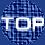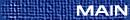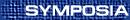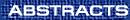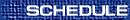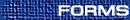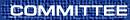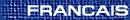Associative Algebras / Algèbres associatives(I. Assem and F. Huard, Organizers) I. ASSEM, Université de Sherbrooke Laura algebras This is a preliminary report on a joint work with Flavio U. Coelho. We define a class of algebras by homological properties, which imply in particular that, over such an algebra, every indecomposable module, except for at most finitely many isomorphism classes, are of projective dimension one or of injective dimension one. We give examples of such algebras, study their properties, give several characterisations as well as a complete description of their Auslander-Reiten quivers and, finally, show that the infinite radical of their module categories are nilpotent of nilpotency index between 3 and 5. J. BILODEAU, Department of Mathematics, University of Toronto, Toronto, Ontario  M5S 3G3 Hochschild cohomology of stable Auslander algebras Let R be a simple singularity and CM(R) the category of maximal Cohen-Macaulay R-modules. It is known that CM(R) is of finite representation type. R. O. Buchweitz showed that in the Kleinian case, the stable Auslander algebra of CM(R) has periodic Hochschild cohomology (of period 2). Comparing the Auslander-Reiten quivers, we explain why the stable Auslander algebra of a simple curve singularity should also have periodic Hoschschild cohomology. J.C. BUSTAMANTE, Université de Sherbrooke, Sherbrooke, Québec  J1k 2R1 Hochschild cohomology and simplicial cohomology of algebras Given a finite dimensional k-algebra A, we compare its Hochschild cohomology groups HHi(A) with its simplicial cohomology groups SHi(A,k). In case A is schurian, it has been shown (Martins-de la Peña) that there is a monomorphism x: SHi(A,k)® HHi(A). We give a sufficient condition for this morphism to be an ismorphism. F. HUARD, Bishop's University, Lennoxville Unit forms of tree algebras Let q be a unit form. It is possible to associate to q a graph Gq with two types of edges (full and dotted). We consider unit forms q such that the full edges of Gq form a connected tree. We give necessary and sufficient conditions for Gq to be the underlying graph of a bound quiver tree algebra A=kQ/I. M. KLEINER, Syracuse University, Syracuse, New York  13244, USA The graded preprojective algebra of a quiver We introduce a new grading on the preprojective algebra of an arbitrary locally finite quiver. Viewing the algebra as a left module over the path algebra, we use the grading and the geometry of paths to construct explicitly a canonical collection of its submodules and of exact sequences involving these submodules. If a vertex of the quiver is a source, the submodules behave nicely with respect to the reflection functor associated to the vertex. If the quiver is finite and without oriented cycles, the canonical submodules are the nonisomorphic indecomposable preprojective modules and the canonical exact sequences are the almost split sequences with preprojective terms. Our proof is inspired by the argument of Gelfand and Ponomarev in the case when the finite quiver is a tree. MARCELO LANZILOTTA, Universidad de la República, Uruguay About weakly shod algebras (Joint work with F. Coelho, IME-USP, Brasil) In this talk we shall begin with a general review of weakly shod algebras, which are defined by the following property: there is a positive integer M such that any path of nonzero morphisms from an indecomposable injective module to an indecomposable projective module has length at most M. The familly of weakly shod algebras contains the classes of tilted algebras, quasitilted algebras and shod algebras. We shall continue talking about some aspects of the ordinary quiver of a weakly shod algebra. Looking forward a classification of such quivers we get some necessary conditions. J. LÉVESQUE, Universit'e de Sherbrook, Sherbrooke, Québec  J1K 2R1 Module inclinant sur un produit fibré À partir de deux K-algèbres A et B (où K est un corps algébriquement clos) qui ont une algèbre quotient C en commun, on peut considérer l'algèbre R qui est le produit fibré des surjections canoniques A ® C et B ® C. Il est possible, de cette façon, de construire une grande variété d'algèbres, et j'étudie celles-ci sous l'angle de l'inclinaison : on voit comment construire un module inclinant sur R à partir d'un module inclinant sur C qui est pré-inclinant sur A et B. SHIPING LIU, Universite de Shebrooke, Sherbrooke, Quebec  J1K 2R1 Mesh algebras without outer derivations (joint work with Ragnar-Olaf Buchweitz) The purpose of this talk is to give a characterisation of mesh algebras over a commutative ring which admit no outer derivation. To be more precise, we shall present the following result. Theorem. Let R(G) be the mesh algebra of a finite translation quiver Gover a commutative ring with an identity R. The following conditions are equivalent are equivalent: (1) HH1R(G) vanishes. (2) Gis simply connected. (3) HH1R(D) vanishes for every convex translation subquiver Dof G. Note that although vanishing of HH1(A) of an algebra A does not in general imply the triangularity of A, it is still interesting to study for which classes of algebras, one has an affirmative answer. Our above result shows that this is the case for mesh algebras. A. MARTSINKOVSKY, Northeastern University, 360 Huntington Ave. Boston, Massachusetts  02115, USA Noncommutative sheaf cohomology over Koszul quiver algebras This is joint work with R. Martinez Villa. We show that noncommutative sheaf cohomology of Koszul modules over a Koszul quiver algebra is functorially isomorphic, under Koszul duality, to Vogel cohomology of the the Koszul-dual modules over the Koszul-dual algebra. If the original algebra is of finite global dimension, then this isomorphism extends to all modules which can be resolved with finitely generated projectives. If, an addition, the algebra is noetherian, then the above statement is true for all finitely generated modules. This includes the classical case of sheaves on projective space. If time permits, I will mention applications to noncommutative algebraic geometry. R. RAPHAEL, Concordia University, Montreal, Quebec Ring epimorphisms and C(X) We first give some background from the theory of epimorphisms in the category of rings. Then we present three projects from on the topic ``epimorphisms and rings of continuous functions''. The individual themes are as follows: (i) When is the epimorphic hull of C(X) itself a C(Y)? (with Macoosh and Woods) (ii) If G(X) is the least von Neumann regular ring of functions on X containing C(X), when is G(X) a C(Y)? (with Henriksen and Woods) (iii) Let X be a topological space and let Y be a subspace of X. Find necessary and sufficient conditions for the induced ring homomorphism C(X)® C(Y) to be a ring epimorphism. (with Burgess). DAVID SMITH, Université de Sherbrooke, Sherbrooke, Québec  J1K 2R1 From simplicial complexes to partially ordered sets and back This is a report on a joint work with J. C. Bustamante and J. Dionne. Our objective is to simplify, in certain cases, the computation of the fundamental groups of simplicial complexes and incidence algebras of posets. For this purpose, we consider the functor O which associates to a simplicial complex its non-degenerate simplices ordered by inclusion, and the functor D which associates to each poset its chain complex. It is known that the composition O°D preserves the fundamental group. We give combinatorial characterisations of the images of O and O°D as well as an algorithm allowing to construct a preimage of any element in the image of O°D.